# Introduction

I worked on a project with my friends using simple reinforcement learning, so I also wrote a record of it in an article.

# background

Many people may have had trouble finding a parking lot in a crowded city. According to one theory, about 30% of the traffic in the city area seems to be wandering to find a parking lot [^ 1]. I thought that it would be possible to alleviate road congestion if the parking lot selection for each vehicle could be instructed (action selection) in real time in consideration of the road conditions (conditions). Therefore, we introduced guide lights that instruct the parking lot to enter the parking lot in real time, and trained them using Deep Q-Network.

# idea

## Past research

As a study of signal control using reinforcement learning Pol and Oliehoek(2016), Coordinated Deep Reinforcement Learners for Traffic Light Control, NIPS'16 Workshop on Learning, Inference and Control of Multi-Agent Systems And so on. In this research, signal control is learned using DQN on a two-dimensional road network, but the design of the reward function is a mistake, and the reward function incorporates congestion, vehicle waiting time, presence or absence of sudden stop, etc. There is.

Reference: Reward function used in previous studies $r_t = -0.1c - 0.1 \sum_{i=1}^N j_i - 0.2 \sum_{i=1}^N e_i - 0.3 \sum_{i=1}^N d_i - 0.3\sum_{i=1}^N w_i$

$i = 1, \ dots, N$: Agent (vehicle) $r$: Reward $c$: Whether the signal has changed $j$: Whether the vehicle was caught in a traffic jam $e$: Whether the vehicle has stopped suddenly $d$: Whether the speed of the vehicle is below a certain speed (speed limit) $w$: Whether the vehicle stopped at a red light

However, this study does not deal with parking and the accompanying pauses that contribute to traffic congestion, as vehicles simply pass through the road. In addition, a simulator called SUMO [^ 2] is used for traffic simulation, which is a little troublesome to handle.

## Ideas for this project

So in this project

1. Focus on vehicle parking behavior and learn parking lot guide lights
2. Introducing cellular automata in the road network and the expression of parking and congestion, making it easier to handle
3. Dynamically control each guide light to reduce parking time while reducing traffic congestion

I implemented it with this in mind.

# model

Environment The environment was expressed using a cellular automaton, assuming a simple one-dimensional road space.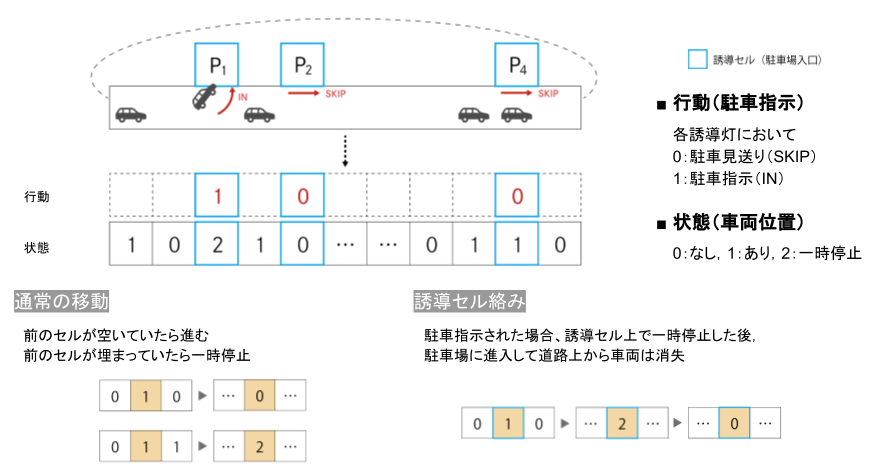The status settings are as shown in the figure above. At t = 0, cars line up in the initial position on the one-dimensional road space. There are several parking lots along the road space, and all cars want to store in one of them. The car enters one of the parking lots while traveling on the road space. Here, parking lots are indiscriminate for all cars, that is, any car can be parked in any parking lot. Each vehicle is warehousing or seeing off according to the instructions (IN / SKIP) of the guide light installed in front of the parking lot.

The state on the cell is 0: No car 1: There is a car (running) 2: Car (paused)

The behavior of the guide lights 0: Send off receipt (SKIP) 1: Goods receipt instruction (IN)

The transition rule is Normal move: Move forward if the previous cell is empty. Pause if there is a car in the previous cell. Guidance cell: When warehousing is instructed, after pausing at that cell, it enters the parking lot and disappears from the road.

Based on the above, the Python code is as follows [^ 3].

#### environment.py


import os
import numpy as np
import random

class Parking:
def __init__(self, test = False):
self.name = os.path.splitext(os.path.basename(__file__))
self.n_cells = 50 # number of cells
self.n_cars = 20 # number of cars at t=0
self.n_parking = 4 # number of parking
self.enable_actions = ([0, 0, 0, 0], [0, 0, 0, 1], [0, 0, 1, 0], [0, 0, 1, 1],
[0, 1, 0, 0], [0, 1, 0, 1], [0, 1, 1, 0], [0, 1, 1, 1],
[1, 0, 0, 0], [1, 0, 0, 1], [1, 0, 1, 0], [1, 0, 1, 1],
[1, 1, 0, 0], [1, 1, 0, 1], [1, 1, 1, 0], [1, 1, 1, 1])
self.pre_action = [0, 0, 0, 0]
self.parking_position = [10, 20, 30, 40]    # fixed parking locations
self.frame_rate = 5
self.rules = [ "000222000000222000000222000", # 111
"000222000000222000000222000", # 110
"220220000220220000220220000", # 101
"220220000220220000220220000", # 100
"000222111000222111000222000", # 011
"000222111000222111000222000", # 010
"220220111220220111220220000", # 001
"220220111220220111220220000"  # 000
]
self.frame_rate = 5
self.test = test

# variables
self.reset()

def update(self, action):
for i, p in enumerate(self.parking_position):

# update car position

# calculate reward
self.reward = 0
self.terminal = False
self.n_remain = self.count_remain(self.car_cells)
self.n_stop = self.count_stop(self.car_cells)
c = np.sum(np.abs(np.array(action) - np.array(self.pre_action))) / self.n_parking

# reward function
self.reward = - self.n_remain/self.n_init - self.n_stop/self.n_init

if self.n_remain == 0:
self.terminal = True

self.pre_action = action

def rule_choice(self, l, x, r):
# decide the transition rule by the parking navigation
bin_num = int(str(l)+str(x)+str(r), 2)
return self.rules[-(bin_num+1)]

def transition(self, l, x, r, rule):
# decide the next state
tri_num = int(str(l)+str(x)+str(r), 3)
return int(rule[-(tri_num+1)])

def count_remain(self, state):
#count remaining cars
return len([i for i in state if i > 0])

def count_stop(self, state):
# count stopping cars
return len([i for i in state if i == 2])

state_new = []

# add 1 cell at the edge for periodic boundary conditions
state = [state[-1]] + state
state.append(state)

# calculate the next state of each cell
for i in range(1, len(state)-1):
state_new.append(self.transition(state[i-1], state[i], state[i+1], rule))

return state_new

def draw(self):
# reset screen
self.screen = np.array(self.car_cells)

def observe(self):
self.draw()
return self.screen, self.reward, self.terminal, self.n_remain, self.n_stop

def execute_action(self, action):
self.update(action)

def reset(self):
random.seed(300) # car position at t=0 is fixed
self.car_cells =  * self.n_cars +  * (self.n_cells - self.n_cars)
random.shuffle(self.car_cells)

self.n_init = len([i for i in self.car_cells if i > 0])
self.n_remain = self.count_remain(self.car_cells)
self.n_stop = self.count_stop(self.car_cells)

# reset other variables
self.reward = 0
self.terminal = False


Agent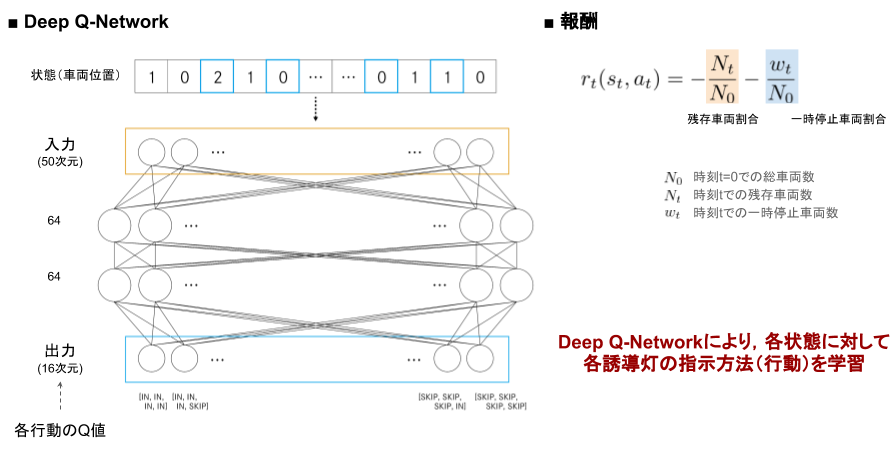The image of Agent learning and reward design is shown in the figure above. The state (vehicle position) is input, and the Q value of the action (combination of guide light instructions) is output with two fully connected layers in between.

The reward is -Ratio of the number of remaining vehicles at time t to the total number of vehicles -Ratio of the number of temporarily stopped vehicles at time t to the total number of vehicles Was used.

The Python code based on the above is as follows.

#### agent.py


from collections import deque
import os
import numpy as np
import tensorflow as tf
# it is necessary if tensorflow version > 2.0
import tensorflow.compat.v1 as tf
tf.disable_v2_behavior()

class DQNAgent:
"""
Multi Layer Perceptron with Experience Replay
"""
def __init__(self, enable_actions, environment_name, n_cells):
# parameters
self.name = os.path.splitext(os.path.basename(__file__))
self.environment_name = environment_name
self.enable_actions = enable_actions
self.n_actions = len(self.enable_actions)
self.minibatch_size = 32
self.replay_memory_size = 1000
self.learning_rate = 0.001
self.discount_factor = 0.9
self.exploration = 0.1
self.model_dir = os.path.join(os.path.dirname(os.path.abspath(__file__)), "models")
self.model_name = "{}.ckpt".format(self.environment_name)
self.n_cells = n_cells

# replay memory
self.D = deque(maxlen=self.replay_memory_size)

# model
self.init_model()

# variables
self.current_loss = 0.0

def init_model(self):
# input layer (1 x n_cells)
self.x = tf.placeholder(tf.float32, [None, self.n_cells])

# flatten (n_cells)
x_flat = tf.reshape(self.x, [-1, self.n_cells])

# fully connected layer 1
W_fc1 = tf.Variable(tf.truncated_normal([self.n_cells, 64], stddev=0.01))
b_fc1 = tf.Variable(tf.zeros())
h_fc1 = tf.nn.relu(tf.matmul(x_flat, W_fc1) + b_fc1)

# fully connected layer 2
W_fc2 = tf.Variable(tf.truncated_normal([64, 64], stddev=0.01))
b_fc2 = tf.Variable(tf.zeros())
h_fc2 = tf.nn.relu(tf.matmul(h_fc1, W_fc2) + b_fc2)

# output layer (n_actions)
W_out = tf.Variable(tf.truncated_normal([64, self.n_actions], stddev=0.01))
b_out = tf.Variable(tf.zeros([self.n_actions]))
self.y = tf.matmul(h_fc2, W_out) + b_out

# loss function
self.y_ = tf.placeholder(tf.float32, [None, self.n_actions])
self.loss = tf.reduce_mean(tf.square(self.y_ - self.y))

# train operation
optimizer = tf.train.RMSPropOptimizer(self.learning_rate)
self.training = optimizer.minimize(self.loss)

# saver
self.saver = tf.train.Saver()

# session
self.sess = tf.Session()
self.sess.run(tf.global_variables_initializer())

def Q_values(self, state):
# Q(state, action) of all actions
return self.sess.run(self.y, feed_dict={self.x: [state]})

def select_action(self, state, epsilon):
if np.random.rand() <= epsilon:
# random
indice = np.random.choice(self.n_actions)
return self.enable_actions[indice]
else:
# max_action Q(state, action)
return self.enable_actions[np.argmax(self.Q_values(state))]

def store_experience(self, state, action, reward, state_1, terminal):
self.D.append((state, action, reward, state_1, terminal))

def experience_replay(self):
state_minibatch = []
y_minibatch = []

# sample random minibatch
minibatch_size = min(len(self.D), self.minibatch_size)
minibatch_indexes = np.random.randint(0, len(self.D), minibatch_size)

for j in minibatch_indexes:
state_j, action_j, reward_j, state_j_1, terminal = self.D[j]
action_j_index = self.enable_actions.index(action_j)

y_j = self.Q_values(state_j)

if terminal:
y_j[action_j_index] = reward_j
else:
# reward_j + gamma * max_action' Q(state', action')
y_j[action_j_index] = reward_j + self.discount_factor * np.max(self.Q_values(state_j_1))  # NOQA

state_minibatch.append(state_j)
y_minibatch.append(y_j)

# training
self.sess.run(self.training, feed_dict={self.x: state_minibatch, self.y_: y_minibatch})

# for log
self.current_loss = self.sess.run(self.loss, feed_dict={self.x: state_minibatch, self.y_: y_minibatch})

if model_path:
self.saver.restore(self.sess, model_path)
else:
checkpoint = tf.train.get_checkpoint_state(self.model_dir)
if checkpoint and checkpoint.model_checkpoint_path:
self.saver.restore(self.sess, checkpoint.model_checkpoint_path)

def save_model(self):
self.saver.save(self.sess, os.path.join(self.model_dir, self.model_name))


# Learning

After learning the model with the settings seen above, conduct an experiment to check how efficiently the vehicle can be controlled compared to the case where it is not learned (= all guide lights indicate warehousing).

The learning and experimental Python code is below.

#### train.py


import numpy as np
from environment import Parking
from agent import DQNAgent

if __name__ == "__main__":
# parameters
n_epochs = 1000

# environment, agent
env = Parking(test = False)
agent = DQNAgent(env.enable_actions, env.name, env.n_cells)

for e in range(n_epochs):
# reset
frame = 0
loss = 0.0
Q_max = 0.0
env.reset()
state_t_1, reward_t, terminal, state_remain, state_stop = env.observe()

while not terminal:
state_t = state_t_1

# execute action in environment
action_t = agent.select_action(state_t, agent.exploration)

env.execute_action(action_t)

# observe environment
state_t_1, reward_t, terminal, state_remain, state_stop = env.observe()

# store experience
agent.store_experience(state_t, action_t, reward_t, state_t_1, terminal)

# experience replay
agent.experience_replay()

# for log
frame += 1
loss += agent.current_loss
Q_max += np.max(agent.Q_values(state_t))

print("EPOCH: {:03d}/{:03d} | FRAME: {:d} | LOSS: {:.4f} | Q_MAX: {:.4f}".format(
e, n_epochs - 1, frame, loss / frame, Q_max / frame))

# save model
agent.save_model()


#### test.py


import matplotlib.pyplot as plt
import argparse
import datetime
import os
from environment import Parking
from agent import DQNAgent
import csv
import pprint

if __name__ == "__main__":
# args
parser = argparse.ArgumentParser()
parser.set_defaults(save=False)
args = parser.parse_args()

# environmet, agent
env = Parking(test = True)
agent = DQNAgent(env.enable_actions, env.name, env.n_cells)

# variables
score = 0
state_t_1, reward_t, terminal, state_remain, state_stop = env.observe()
result_state = []
result_remain = []
result_stop = []
n_cars = state_remain

while not terminal:
result_state.append(list(state_t_1))
result_remain.append(state_remain)
result_stop.append(state_stop)
state_t = state_t_1

# execute action in environment
action_t = agent.select_action(state_t, 0.0)
else:
action_t = [1,1,1,1]

env.execute_action(action_t)
state_t_1, reward_t, terminal, state_remain, state_stop = env.observe()

result_remain.append(state_remain)
result_stop.append(state_stop)

print("conversion time = " + str(len(result_remain)))   # total time
print("average time = " + str(sum(result_remain) / n_cars)) # average parking time
print("extra stopping time = " + str(sum(result_stop) - n_cars)) # extra stopping time

# output for csv
with open('output/result.csv', 'a') as f:
writer = csv.writer(f)
writer.writerow([len(result_remain), sum(result_remain) / n_cars, sum(result_stop) - n_cars])

# mkdir for output
dirname_n_remain = "output/figure/n_remain/"
dirname_n_stop = "output/figure/n_stop/"
dirname_state = "output/figure/state/"

os.makedirs(dirname_n_remain, exist_ok=True)
os.makedirs(dirname_n_stop, exist_ok=True)
os.makedirs(dirname_state, exist_ok=True)
# file name is date+time
now = datetime.datetime.now()

n_remain_filename = dirname_n_remain + now.strftime('%Y%m%d_%H%M%S') + '.png'
n_stop_filename = dirname_n_stop + now.strftime('%Y%m%d_%H%M%S') + '.png'
state_filename = dirname_state + now.strftime('%Y%m%d_%H%M%S') + '.png'

# make the graph of number of remaining cars
epoch = range(len(result_remain))
plt.figure()
plt.xlabel("time")
plt.ylabel("number of remained cars")
plt.plot(epoch, result_remain);
plt.show()

# make the graph of stopping cars
epoch = range(len(result_stop))
plt.figure()
plt.xlabel("time")
plt.ylabel("number of stopping cars")
plt.plot(epoch, result_stop);
plt.show()

# make the figure of state transition
plt.figure()
plt.title("State Transition")
plt.imshow(result_state, cmap="binary")
plt.show()


# result

### Changes in the number of remaining vehicles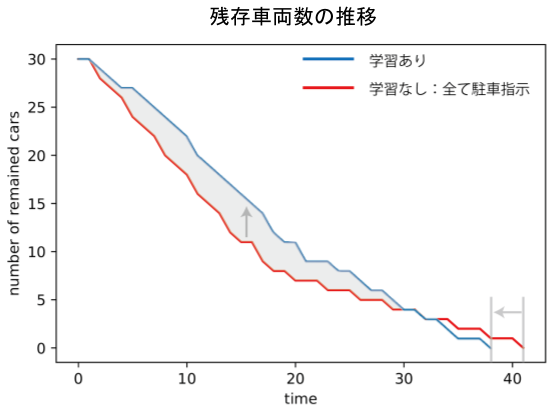The transition of the number of remaining vehicles is as shown in the above figure (initial number of vehicles 30). After learning, the number of cars did not decrease at the initial stage, but the time required for all cars to park was shortened. This is due to the fact that some cars are instructed to "dare to see off" in order to prevent traffic congestion that occurs when the parking lot is biased toward a specific parking lot. It is a form that is socially optimized at the expense of the profits of some vehicles.

### State transition (initial number of vehicles 30)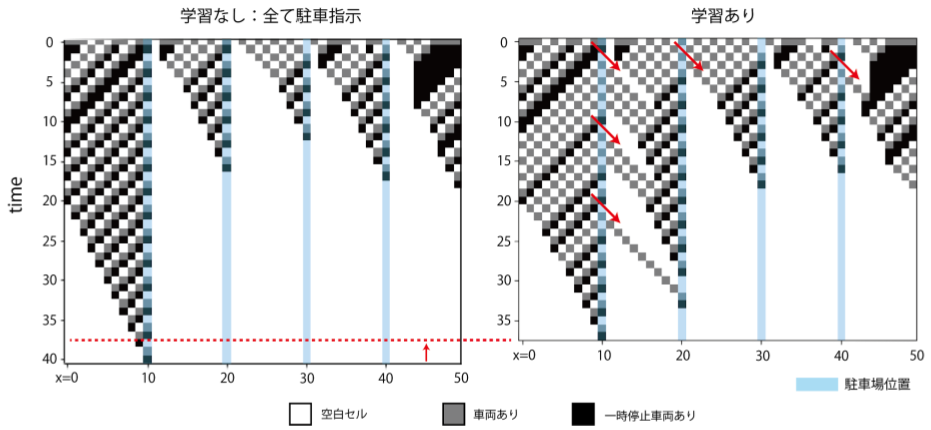Let's take a look at the state transition to see what the actual movement of the vehicle is. The vertical axis direction is time, and the horizontal axis direction is each time. Given the initial state at t = 0, the state changes when time-evolved are accumulated downward.

The state transition when the initial number of vehicles is 30 is as shown in the above figure. As seen in the transition of the number of remaining vehicles, by giving instructions to "dare to see off" some vehicles, future traffic congestion will be eased, and as a result, parking of all vehicles will be completed earlier.

### State transition (initial number of vehicles 20)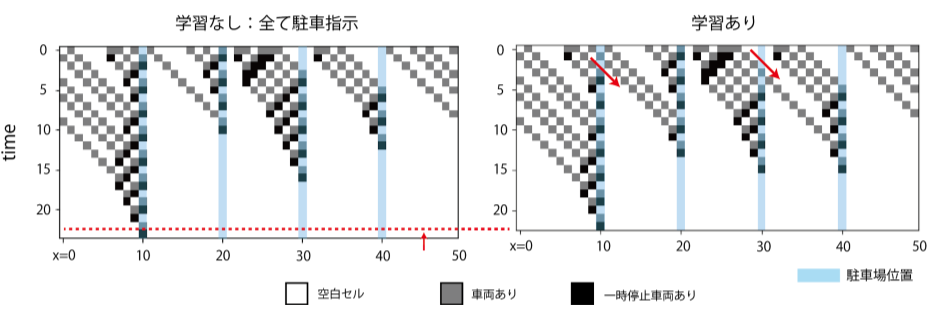The state transition when the initial number of vehicles is 20 is as shown in the above figure. As in the case of the initial number of vehicles of 30, by instructing "dare to see off", future traffic congestion will be alleviated, and as a result, parking of all vehicles will be completed earlier. From this, it can be seen that learning is robust to some extent with respect to the number of vehicles.

### State transition (initial number of vehicles 10)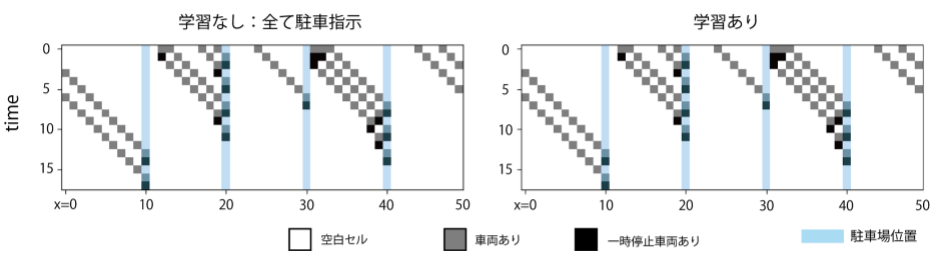The state transition when the initial number of vehicles is 10 is as shown in the above figure. If the number of initial vehicles is small, traffic congestion will not occur and it is judged that there is no need to give an instruction to "dare to see off".

# Conclusion

-A simple description of the road space with parking behavior using a cellular automaton.

-The strategy of "putting in the parking lot you find for the time being" may increase the time it takes for all vehicles to complete parking.

・ By introducing guide lights that instruct parking lots and learning using DQN, it is possible to shorten the time it takes for all vehicles to complete parking.